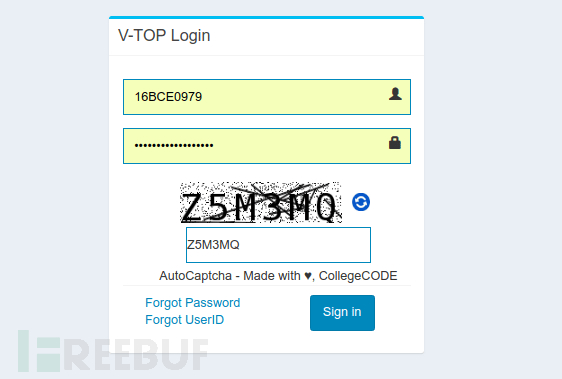``````from PIL import Image
image = Image.open("filename.xyz")
cropped_image = image.crop((left, upper, right, lower))``````

``````from PIL import Image
image = Image.open("captcha.png").convert("L") # Grayscale conversion cropped_image = image.crop((0, 0, 30, 45))
cropped_image.save("cropped_image.png")``````

``````pixel_matrix = cropped_image.load() for col in range(0, cropped_image.height): for row in range(0, cropped_image.width): if pixel_matrix[row, col] != 0:
pixel_matrix[row, col] = 255 image.save("thresholded_image.png")``````

``````for column in range(1, image.height - 1): for row in range(1, image.width - 1): if pixel_matrix[row, column] == 0 \ and pixel_matrix[row, column - 1] == 255 and pixel_matrix[row, column + 1] == 255 :
pixel_matrix[row, column] = 255 if pixel_matrix[row, column] == 0 \ and pixel_matrix[row - 1, column] == 255 and pixel_matrix[row + 1, column] == 255:
pixel_matrix[row, column] = 255``````

``````import json
characters = "123456789abcdefghijklmnpqrstuvwxyz" captcha = "" with open("bitmaps.json", "r") as f:
bitmap = json.load(f) for j in range(image.width/6, image.width + 1, image.width/6):
character_image = image.crop((j - 30, 12, j, 44))
matches = {} for char in characters:
match = 0 black = 0 bitmap_matrix = bitmap[char] for y in range(0, 32): for x in range(0, 30): if character_matrix[x, y] == bitmap_matrix[y][x] and bitmap_matrix[y][x] == 0:
match += 1 if bitmap_matrix[y][x] == 0:
black += 1 perc = float(match) / float(black)
matches.update({perc: char.upper()}) try: